SPSS TUTORIALS BASICS ANOVA REGRESSION FACTOR CORRELATION

# SPSS Kolmogorov-Smirnov Test for Normality

An alternative normality test is the Shapiro-Wilk test.## What is a Kolmogorov-Smirnov normality test?

The Kolmogorov-Smirnov test examines if scores
are likely to follow some distribution in some population.
For avoiding confusion, there's 2 Kolmogorov-Smirnov tests:

• there's the one sample Kolmogorov-Smirnov test for testing if a variable follows a given distribution in a population. This “given distribution” is usually -not always- the normal distribution, hence “Kolmogorov-Smirnov normality test”.
• there's also the (much less common) independent samples Kolmogorov-Smirnov test for testing if a variable has identical distributions in 2 populations.

In theory, “Kolmogorov-Smirnov test” could refer to either test (but usually refers to the one-sample Kolmogorov-Smirnov test) and had better be avoided. By the way, both Kolmogorov-Smirnov tests are present in SPSS.

## Kolmogorov-Smirnov Test - Simple Example

So say I've a population of 1,000,000 people. I think their reaction times on some task are perfectly normally distributed. I sample 233 of these people and measure their reaction times.
Now the observed frequency distribution of these will probably differ a bit -but not too much- from a normal distribution. So I run a histogram over observed reaction times and superimpose a normal distribution with the same mean and standard deviation. The result is shown below.The frequency distribution of my scores doesn't entirely overlap with my normal curve. Now, I could calculate the percentage of cases that deviate from the normal curve -the percentage of red areas in the chart. This percentage is a test statistic: it expresses in a single number how much my data differ from my null hypothesis. So it indicates to what extent the observed scores deviate from a normal distribution.
Now, if my null hypothesis is true, then this deviation percentage should probably be quite small. That is, a small deviation has a high probability value or p-value.
Reversely, a huge deviation percentage is very unlikely and suggests that my reaction times don't follow a normal distribution in the entire population. So a large deviation has a low p-value. As a rule of thumb, we reject the null hypothesis if p < 0.05. So if p < 0.05, we don't believe that our variable follows a normal distribution in our population.

## Kolmogorov-Smirnov Test - Test Statistic

So that's the easiest way to understand how the Kolmogorov-Smirnov normality test works. Computationally, however, it works differently: it compares the observed versus the expected cumulative relative frequencies as shown below.The Kolmogorov-Smirnov test uses the maximal absolute difference between these curves as its test statistic denoted by D. In this chart, the maximal absolute difference D is (0.48 - 0.41 =) 0.07 and it occurs at a reaction time of 960 milliseconds. Keep in mind that D = 0.07 as we'll encounter it in our SPSS output in a minute.

## The Kolmogorov-Smirnov test in SPSS

There's 2 ways to run the test in SPSS:

• NPAR TESTS as found under AnalyzeNonparametric TestsLegacy Dialogs1-Sample K-S... is our method of choice because it creates nicely detailed output.
• EXAMINE VARIABLES from AnalyzeDescriptive StatisticsExplore is an alternative. This command runs both the Kolmogorov-Smirnov test and the Shapiro-Wilk normality test.
Note that EXAMINE VARIABLES uses listwise exclusion of missing values by default. So if I test 5 variables, my 5 tests only use cases which don't have any missings on any of these 5 variables. This is usually not what you want but we'll show how to avoid this.

We'll demonstrate both methods using speedtasks.sav throughout, part of which is shown below.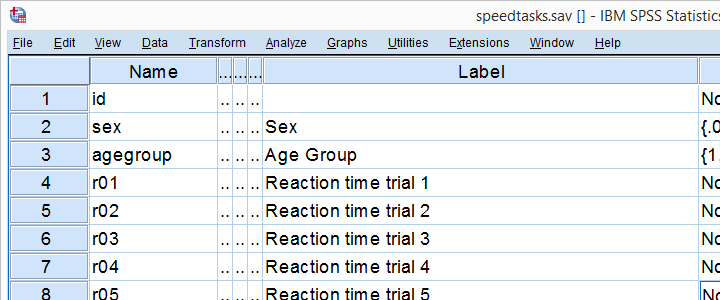Our main research question is which of the reaction time variables is likely
to be normally distributed in our population?
These data are a textbook example of why you should thoroughly inspect your data before you start editing or analyzing them. Let's do just that and run some histograms from the syntax below.

*Run basic histograms for inspecting if distributions look plausible.

frequencies r01 to r05
/format notable
/histogram normal.

*Note that some distributions do not look plausible at all!

## Result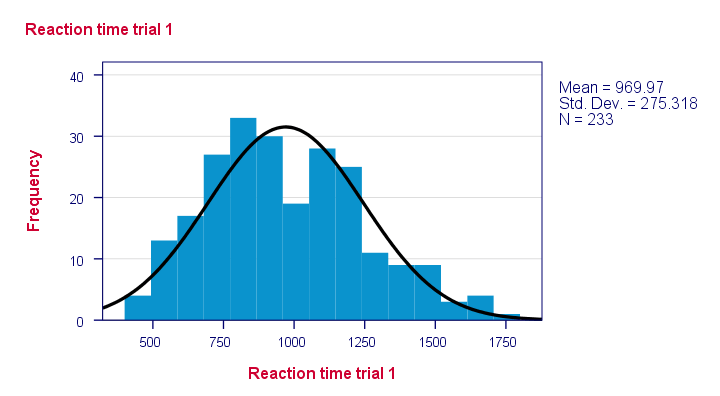Note that some distributions do not look plausible at all. But which ones are likely to be normally distributed?

## SPSS Kolmogorov-Smirnov test from NPAR TESTS

Our preferred option for running the Kolmogorov-Smirnov test is under AnalyzeNonparametric TestsLegacy Dialogs1-Sample K-S... as shown below.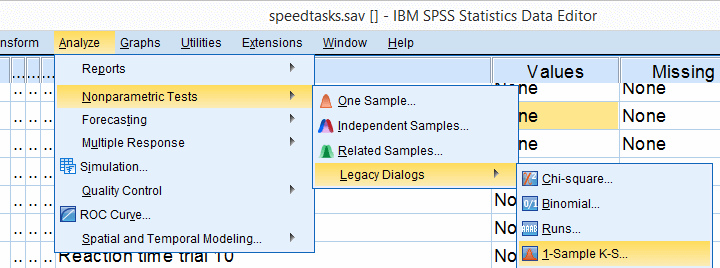Next, we just fill out the dialog as shown below.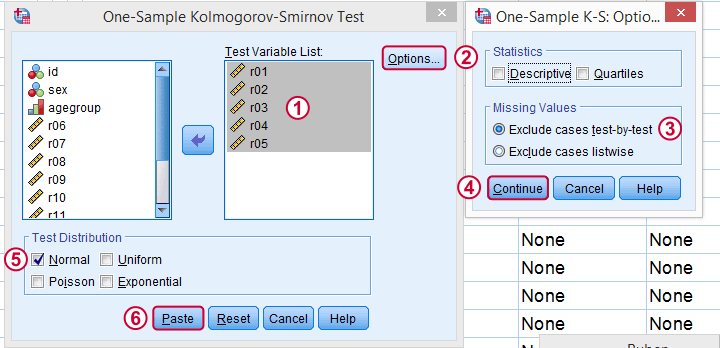Clicking results in the syntax below. Let's run it.

## Kolmogorov-Smirnov Test Syntax from Nonparametric Tests

*One-sample Kolmogorov-Smirnov test from analyze - nonparametric tests - legacy dialogs - 1 sample ks-test.

NPAR TESTS
/K-S(NORMAL)=r01 r02 r03 r04 r05
/MISSING ANALYSIS.

*Only reaction time 4 has p > 0.05 and thus seems normally distributed in population.

## ResultsFirst off, note that the test statistic for our first variable is 0.073 -just like we saw in our cumulative relative frequencies chart a bit earlier on. The chart holds the exact same data we just ran our test on so these results nicely converge.
Regarding our research question: only the reaction times for trial 4 seem to be normally distributed.

## SPSS Kolmogorov-Smirnov test from EXAMINE VARIABLES

An alternative way to run the Kolmogorov-Smirnov test starts from AnalyzeDescriptive StatisticsExplore as shown below.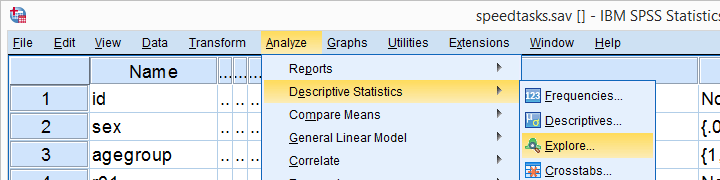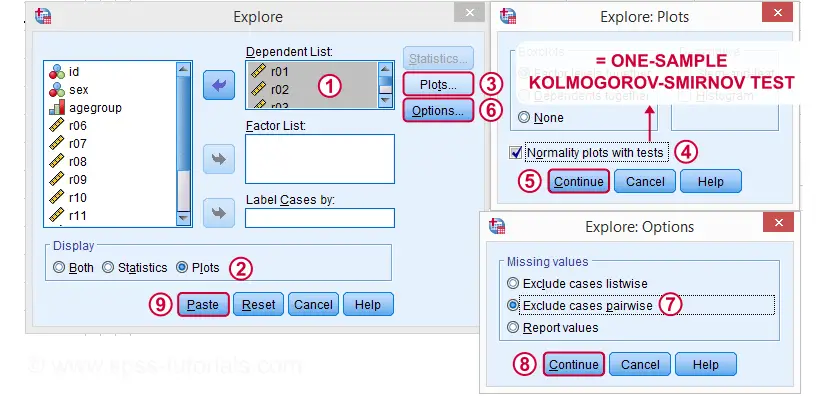## Kolmogorov-Smirnov Test Syntax from Nonparametric Tests

*One-sample Kolmogorov-Smirnov test from analyze - descriptive statistics - explore.

EXAMINE VARIABLES=r01 r02 r03 r04 r05
/PLOT BOXPLOT NPPLOT
/COMPARE GROUPS
/STATISTICS NONE
/CINTERVAL 95
/MISSING PAIRWISE /*IMPORTANT!*/
/NOTOTAL.

*Shorter version.

EXAMINE VARIABLES r01 r02 r03 r04 r05
/PLOT NPPLOT
/missing pairwise /*IMPORTANT!*/.

## ResultsAs a rule of thumb, we conclude that a variable is not normally distributed if “Sig.” < 0.05. So both the Kolmogorov-Smirnov test as well as the Shapiro-Wilk test results suggest that only Reaction time trial 4 follows a normal distribution in the entire population.
Further, note that the Kolmogorov-Smirnov test results are identical to those obtained from NPAR TESTS.

## Reporting a Kolmogorov-Smirnov Test

For reporting our test results following APA guidelines, we'll write something like “a Kolmogorov-Smirnov test indicates that the reaction times on trial 1 do not follow a normal distribution, D(233) = 0.07, p = 0.005.” For additional variables, try and shorten this but make sure you include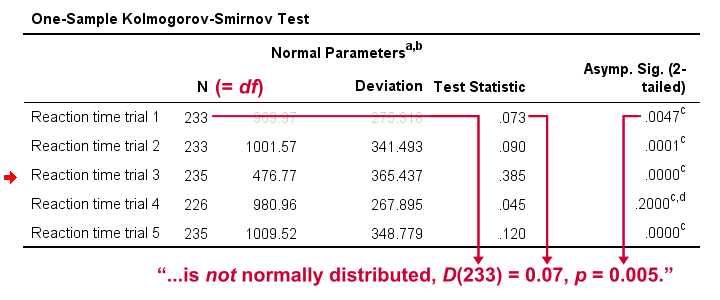## Wrong Results in SPSS?

If you're a student who just wants to pass a test, you can stop reading now. Just follow the steps we discussed so far and you'll be good.

Right, now let's run the exact same tests again in SPSS version 18 and take a look at the output.In this output, the exact p-values are included and -fortunately- they are very close to the asymptotic p-values. Less fortunately, though, the SPSS version 18 results are wildly different
from the SPSS version 24 results
we reported thus far.
The reason seems to be the Lilliefors significance correction which is applied in newer SPSS versions. The result seems to be that the asymptotic significance levels differ much more from the exact significance than they did when the correction is not implied. This raises serious doubts regarding the correctness of the “Lilliefors results” -the default in newer SPSS versions.
Converging evidence for this suggestion was gathered by my colleague Alwin Stegeman who reran all tests in Matlab. The Matlab results agree with the SPSS 18 results and -hence- not with the newer results.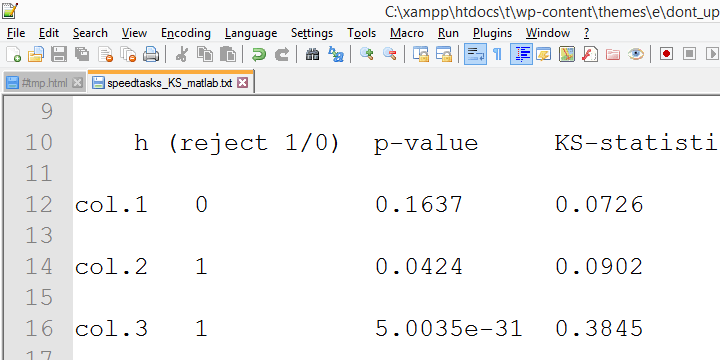## Kolmogorov-Smirnov normality test - Limited Usefulness

The Kolmogorov-Smirnov test is often to test the normality assumption required by many statistical tests such as ANOVA, the t-test and many others. However, it is almost routinely overlooked that such tests are robust against a violation of this assumption if sample sizes are reasonable, say N ≥ 25.The underlying reason for this is the central limit theorem. Therefore, normality tests are only needed for small sample sizes if the aim is to satisfy the normality assumption.
Unfortunately, small sample sizes result in low statistical power for normality tests. This means that substantial deviations from normality will not result in statistical significance. The test says there's no deviation from normality while it's actually huge. In short, the situation in which normality tests are needed -small sample sizes- is also the situation in which they perform poorly.

# Tell us what you think!

*Required field. Your comment will show up after approval from a moderator.

# THIS TUTORIAL HAS 23 COMMENTS:

•### By Jon K Peck on August 27th, 2018

There is a lot to say here.
First, to reduce confusion, you might want to point out that some of the output shown has been transposed from the procedure output as produced.

Second, and more important, the KS test should not be used with estimated parameters without the Lilliefors correction, because the estimated parameters bring the sample distribution closer to the theoretical distribution and that is not accounted for in the significance calculation without this correction.

This difference would not matter asymptotically, but would in finite samples. I tested a few of the SPSS Statistics results against online calculators and got consistent results when including Lliefors.

Third, the KS test for normality with Lliefors has very low power and is inferior to other tests. Shapiro-Wilks is generally recommended over this.

Fourth, another way to test the distribution of the data against various theoretical distributions is to use the Simulation procedure (Analyze > Simulation). Choose Create Simulated Data. On the next panel, select the variables to test. On the Simulation tab, click Fit All.

This will find the best fitting distribution for each variable and show a histogram or bar chart along with the fitted distribution. Select a variable and click Fit Details to see how each candidate distribution fits.

The tests are based on the Anderson-Darling test, but the KS results are also shown.

Finally, it should be noted that one shouldn't be too worried about moderate departures from normality for typical statistical procedures.

•### By Ruben Geert van den Berg on August 28th, 2018

Hi Jon, thanks for your feedback!

Yes, perhaps I should throw in a note about transposing these tables, adjusting decimal places and cleaning up the output.

"...the estimated parameters bring the sample distribution closer to the theoretical distribution..."

I don't see why because my theoretical distribution is a normal distribution with unknown mean and SD. I thought this is the reason why df = N for this test.

Second, if the exact results differ a lot from the asymptotic ones, then either method (or both) must get it wrong, right? If the Liliefors results are accurate, then are the exact results inaccurate?

In any case, I'm no fan of the KS-test at all. I mainly wrote the tutorial because my students are often required to run the test, presumably because some supervisors don't understand the central limit theorem and its implications. The main goal is to help my students pass such tests as easily as possible.

I might acually cover the Shapiro-Wilk test as well at some point but I first have some (a lot of) other things to write about.

The simulation procedure sounds super interesting, I'll definitely try it some time.

•### By Mangala De Zoysa on October 5th, 2018

Very Useful

•### By Hayazi on April 26th, 2019

Hi, I just came across of this test and looking for the information about the practical use of this test. What is the difference of this test result (benefit) to the other Normality test result. Have you any example of the usage of the test practically, used to resolve an issue that you can share.

Thanks

•### By Tengku Arif on July 15th, 2019

Thanks a lot, this website is very useful. Its help me to do a research?